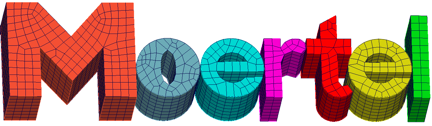Welcome to the Moertel package home page

This package supplies capabilities for nonconforming mesh tying and contact formulations in 2 and 3 dimensions using Mortar methods. Mortar methods are a type of Lagrange Multiplier constraint that can be used in contact formulations and in non-conforming or conforming mesh tying as well as in domain decomposition techniques. Originally introduced as a domain decomposition method for spectral elements, Mortar methods are used in a large class of nonconforming situations such as the surface coupling of different physical models, discretization schemes or non-matching triangulations along interior interfaces of a domain.

Suitable conditions at interfaces are formulated as weak continuity conditions in dual variables introducing Lagrange multipliers on the interface. By an appropriate choice of the function space of the Lagrange multipliers on an interface it is possible to locally condense Lagrange multipliers such that a sparse and definite system of equations can be formed which is equivalent to the saddle point system resulting from the Lagrange multiplier formulation. Moreover if the problems on subdomains are symmetric positive definite (SPD) or symmetric positive indefinite and the complete problem is known to be SPD, then a SPD system of equations can be recovered that leads to the same solution as the saddle point system of equations. This way, unmodified standard solution techniques such as multigrid can be applied and the reduction in the solution costs can be expected to over-compensate for the costs of the Mortar formulation of the problem.